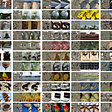# Understanding the Effective Receptive Field in Deep Convolutional Neural Networks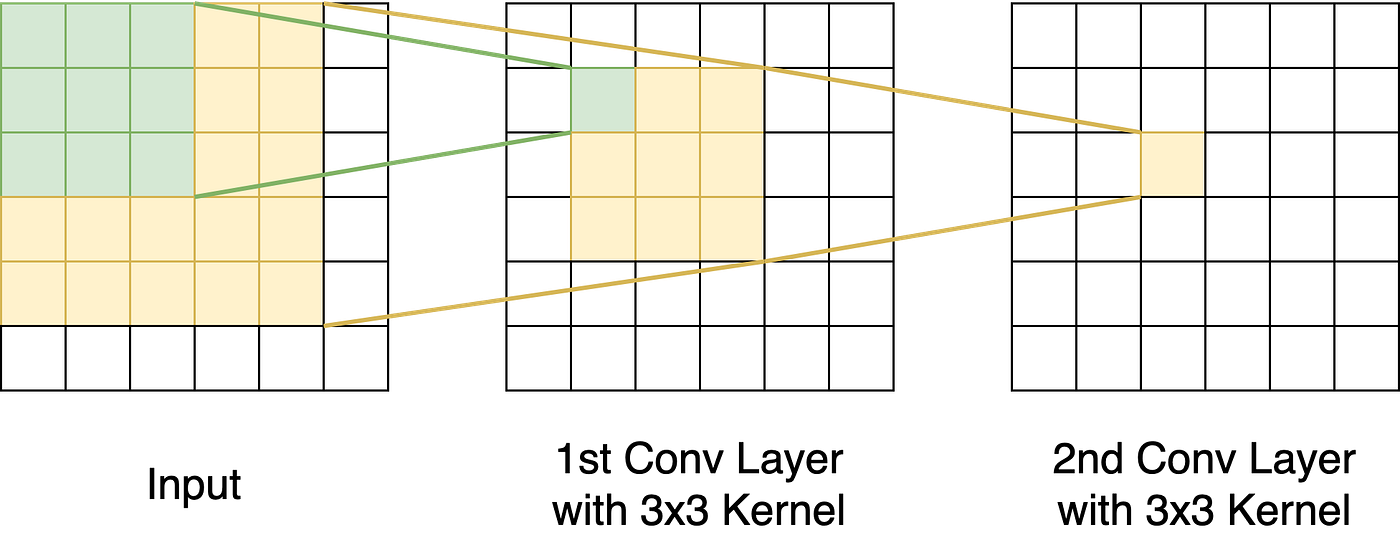Figure 1: The receptive field in a convolutional neural network with two 3x3 convolutional (conv) layers. In the 2nd conv layer, every pixel has a 5x5 field of view, a.k.a. receptive field.

In deep networks, a receptive field — or field of view — is the region in the input space that affects the features of a particular layer as shown in Fig.1. The receptive field is important for understanding and diagnosing a network’s performance. A deep networks should be designed with a receptive field that covers the entire relevant image region because the network is oblivious to regions outside its receptive field.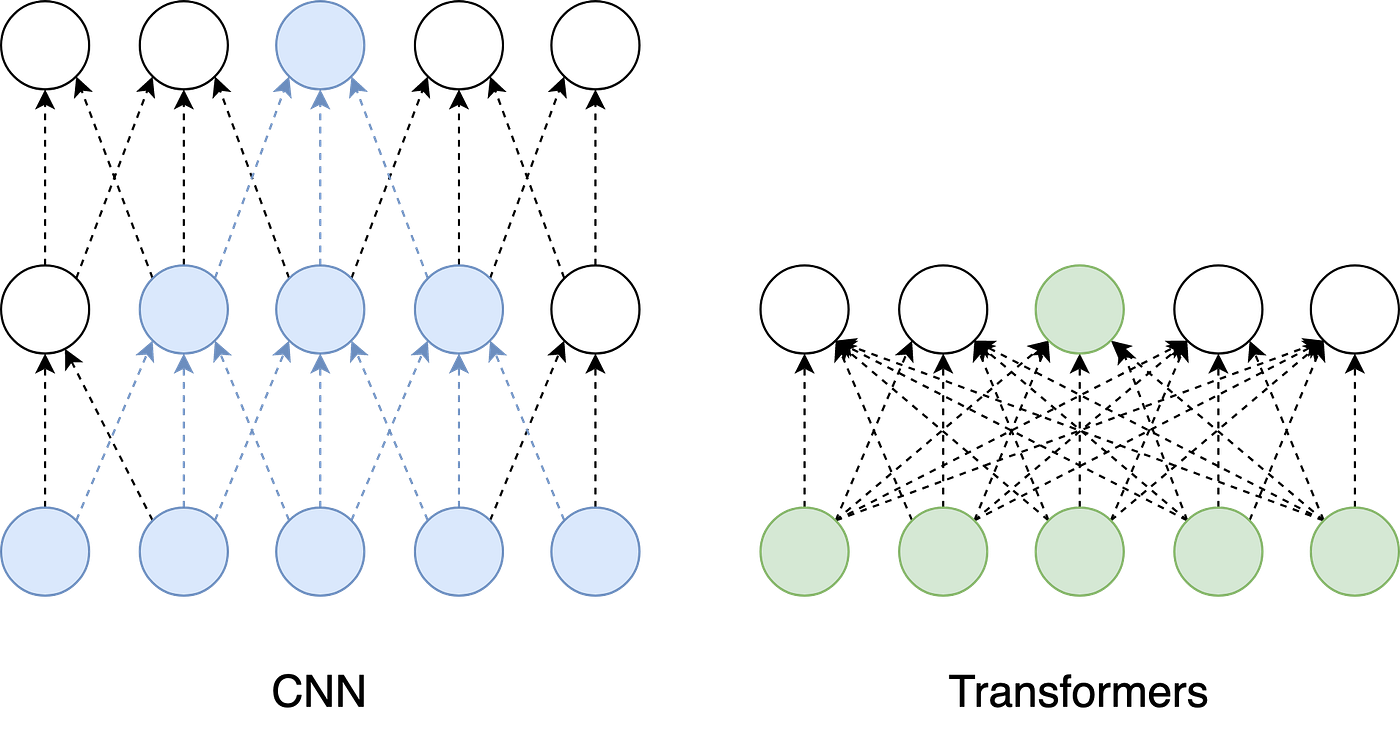Figure 2: Receptive fields of CNNs vs. Transformers. In CNNs, the receptive field grows incrementally one layer after another. In transformers, the receptive field spans all input (tokens) after a single layer. Yet, These receptive fields’ estimates are only theoretical. In CNNs, the actual receptive field differs from the theoretical.

Different network architectures have different receptive fields. Assuming shallow architectures, convolutional neural networks (CNNs) have a smaller receptive field compared to transformers as shown in Fig. 2. In CNNs, the receptive field grows incrementally one layer after another. In transformers, however, the receptive field spans all input (tokens) after a single layer. Yet, these receptive fields’ estimates are only theoretical!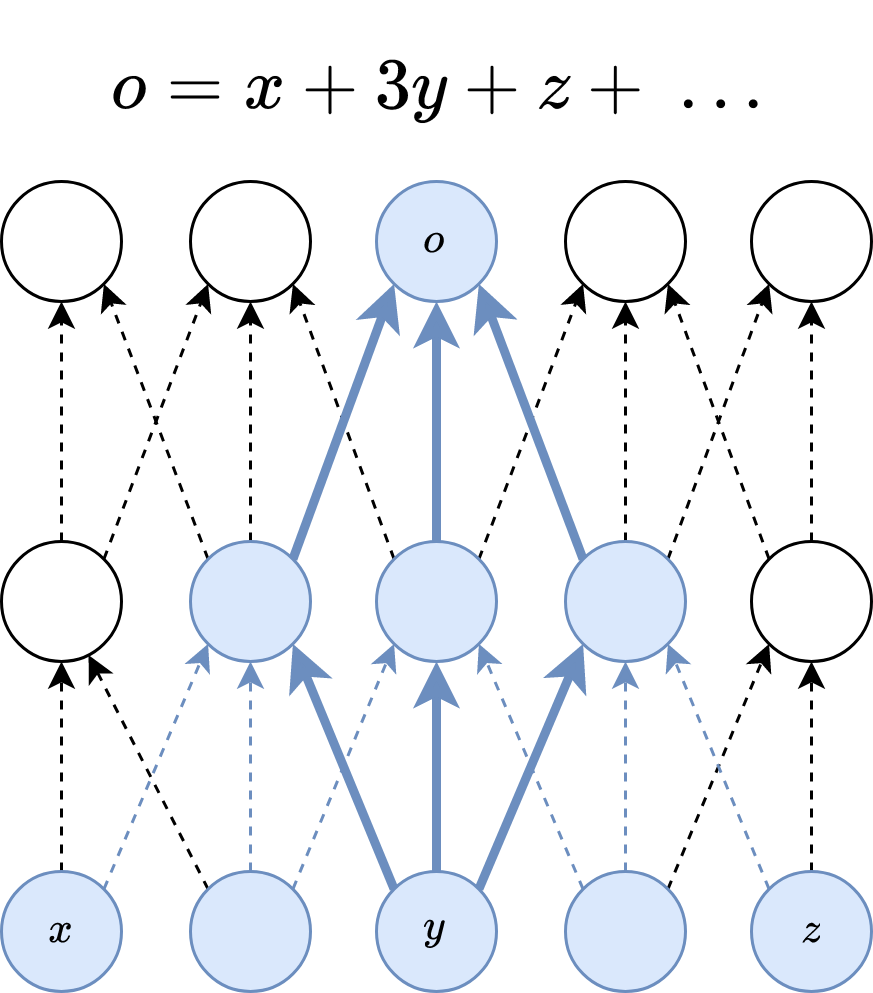Figure 3: In the forward pass, the center pixels can propagate information to the output through many different paths. Therefore, during a backward pass, the center pixels have a much larger gradient magnitude.

In CNNs, the pixels at the center of a receptive field have a large impact on the output. In the forward pass, the center pixels can propagate information to the output through many different paths, while boundary pixels have very few paths to propagate their values as shown in Fig. 3. Therefore, during a backward pass, the center pixels have a much larger gradient magnitude from that output. In this paper , Luo et al. evaluate the receptive field in CNNs empirically and coin the term effective receptive field (ERF).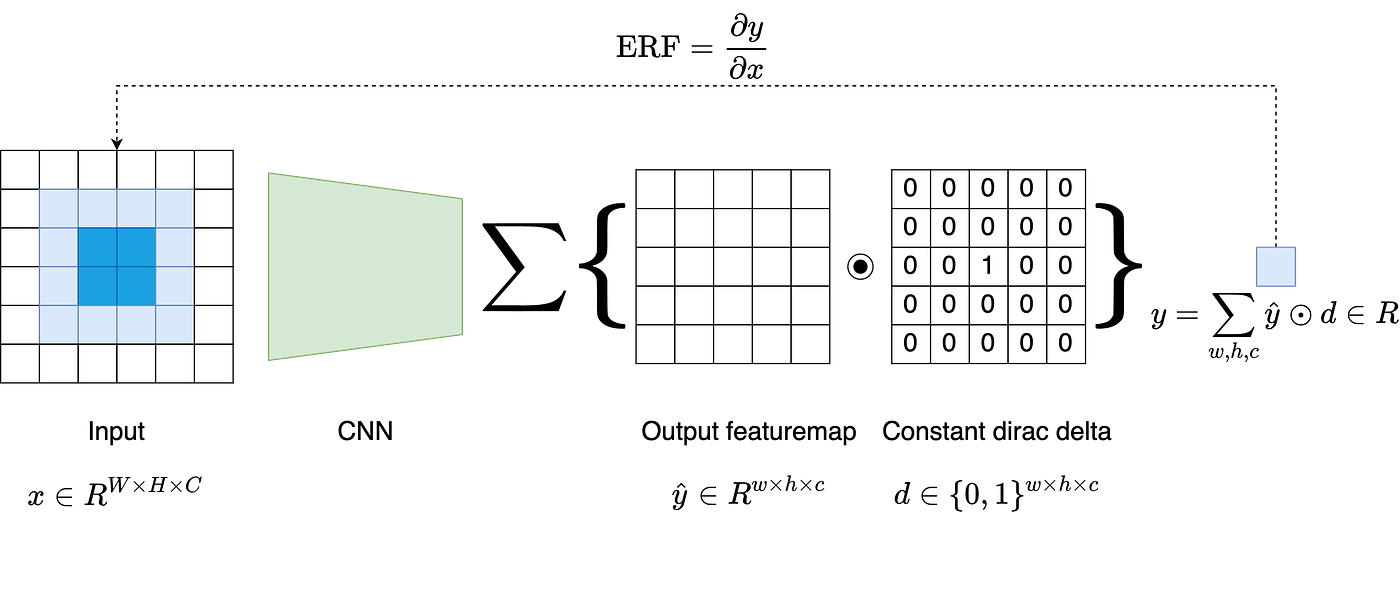Figure 4: The effective receptive field (ERF) is computed using the center pixel in the output feature maps.

Luo et al. show that the ERF both follows a Gaussian distribution and occupies only a fraction of the full theoretical receptive field (TRF). To evaluate the ERF, the paper computes the gradient of the output feature map w.r.t. a given input. To compute the ERF, the multi-dimensional output feature map is reduced into a scalar using a constant Dirac delta as shown in Fig. 4. Basically, the ERF is computed using the center pixel in the output feature map.

While the TRF depends on the architecture only, the ERF dy/dx is dependent on the input, i.e., different inputs generate different ERFs dy/dx. Thus, a single ERF computation is not enough. Accordingly, Luo et al. average the ERF over 20 runs (inputs). Initially, the paper evaluates the ERF using randomly initialized networks. These networks are initialized either uniformly (all ones) or randomly. Once initialized, these networks are fixed to compute the average ERF over 20 runs (inputs).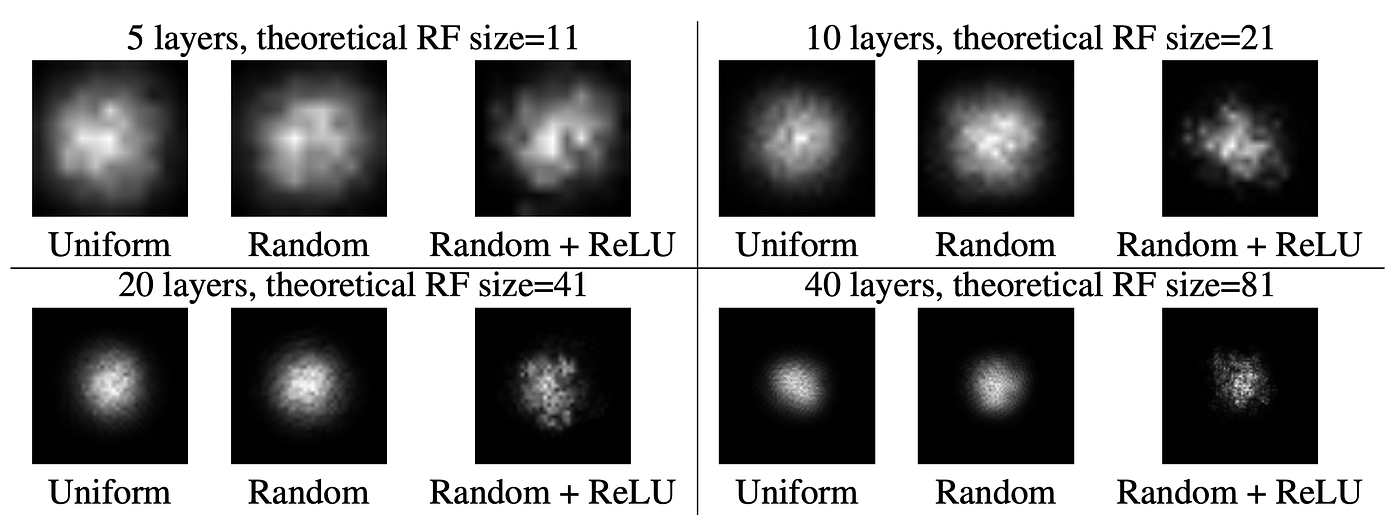Figure 5: Comparing the effect of (1) the number of layers, (2) random weight initialization, and (3) nonlinear activation on the ERF. Kernel size is fixed at 3 × 3 for all the networks. Uniform: convolutional kernel weights are all ones, no nonlinearity; Random: random kernel weights, no nonlinearity; Random + ReLU: random kernel weights, ReLU nonlinearity.

Fig. 5 shows perfect Gaussian shapes for uniformly and randomly initialized convolution kernels without nonlinear activations. Also, the figure shows near Gaussian shapes for randomly weighted kernels with a RELU nonlinearity. Adding the ReLU nonlinearity makes the distribution a bit less Gaussian. ReLU produces exactly zero for half of its inputs and it is easy to get a zero output. This means few paths from the receptive field reach the output.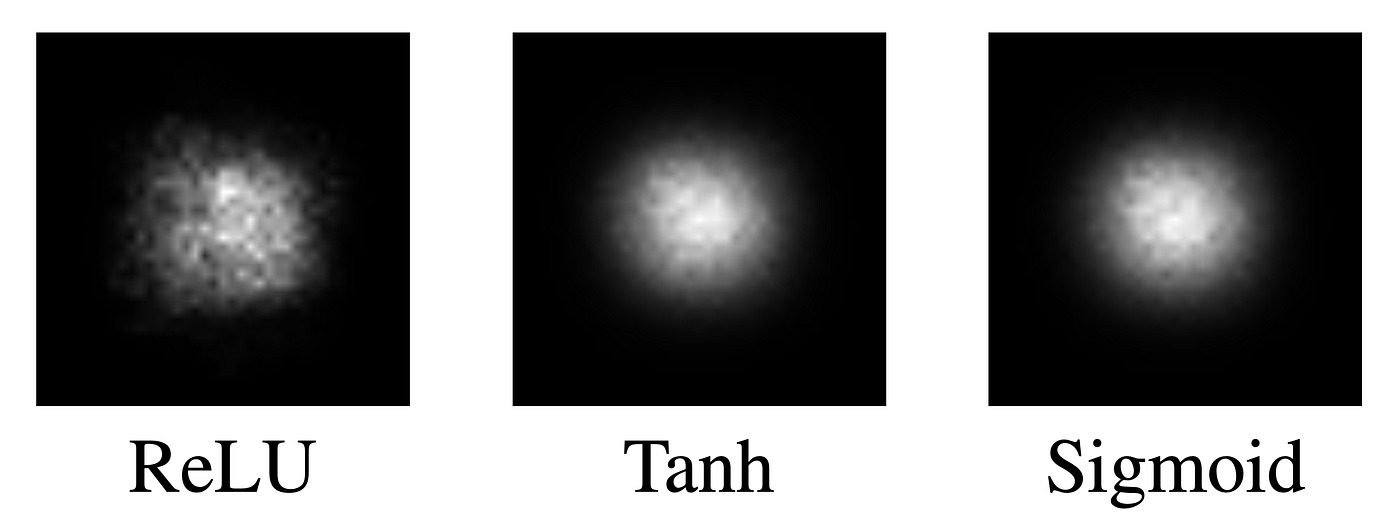Figure 6: Comparing the effect of non-linearities (RELU, Tanh, and Sigmoid) on the ERF. ReLU makes the distribution a bit less Gaussian. ReLU units output exactly zero for half of its inputs. Thus, it is easy to get a zero output for the center pixel on the output plane

Fig. 6 shows the ERF for randomly initialized 20-layer networks. These three networks leverage different non-linearities: RELU, Tanh, and Sigmoid. The ERF is averaged over 100 runs (input) with different random weights as well as different random inputs. Fig. 6 shows the ERF a lot more Gaussian-like.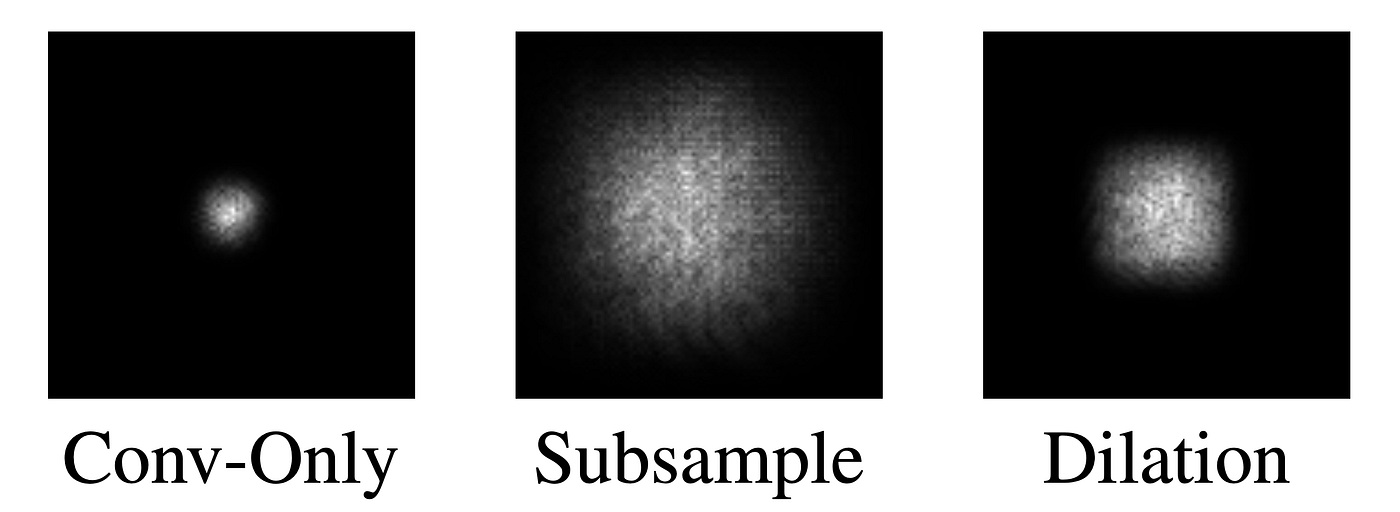Figure 7: Comparing the effect of subsampling and dilation on the ERF. Both increase the ERF significantly.

In computer vision literature, it is typical to use subsampling to reduce the feature resolution as the network depth increases. This paper highlights the importance of both subsampling and dilated-convolutions to increase the ERF. Fig. 7 shows how subsampling and dilated convolution increase the ERF significantly.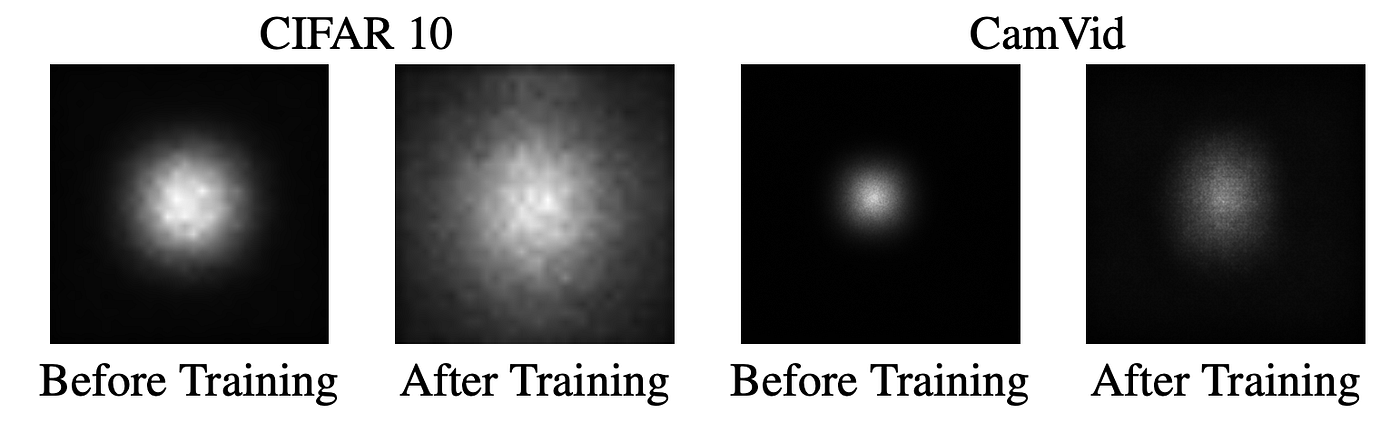Figure 8: Comparison of ERF before and after training for models trained on CIFAR-10 classification and CamVid semantic segmentation tasks.

Fig. 8. evaluates the receptive field before and after training CNNs. The effective receptive field grows significantly after training. In the CIFAR experiment (Fig. 8 left), the theoretical receptive field is 74x74, i.e., bigger than the input image 32x32. Yet, ERF still won’t cover the input image.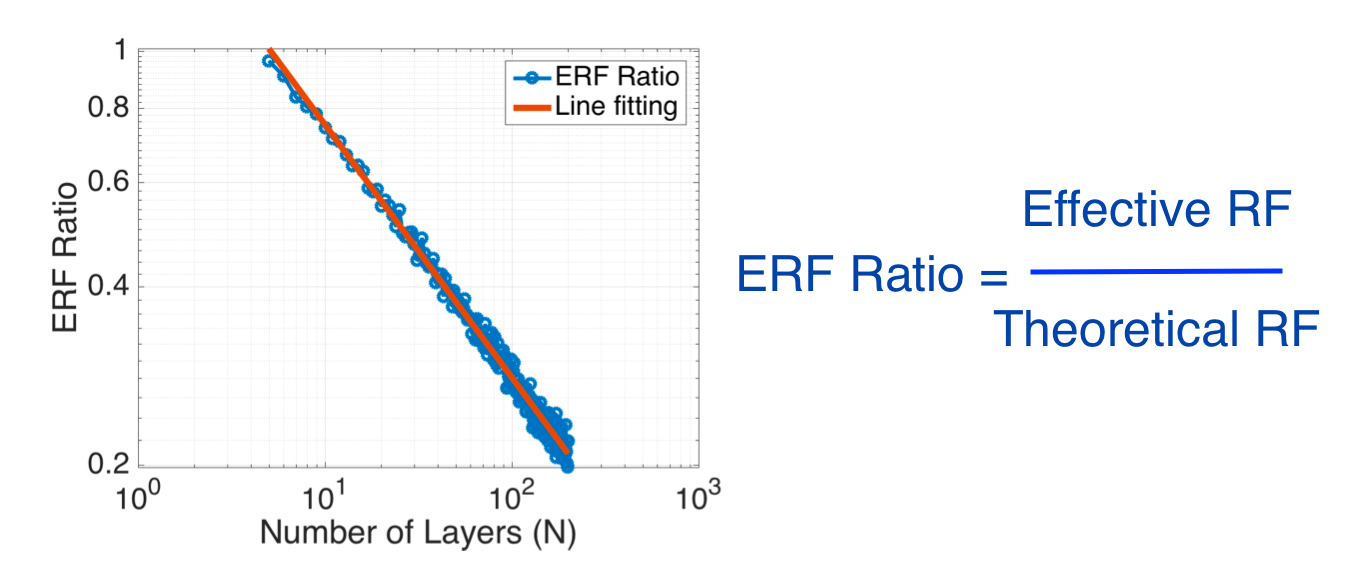Figure 9: The best fitting line for ERF ratio gives a slope -0.43. As the number of layers increases, the effective receptive field decreases w.r.t. theoretical receptive field.

Finally, the paper concludes with an informal relationship between the effective and theoretical receptive fields. The paper fits a line between the number of layers (x-axis) and ERF-ratio (y-axis). Fig. 9 shows the ERF ratio (ERF/TRF) has a -0.43 slope with the number of layers (x-axis). This finding is informal because it is architecture depended. While a negative slope is always expected, the slope value will differ from one architecture to another.

• [S] This is a nice paper and especially important for fields that process high-resolution inputs.
• [S] The paper delivers impressive quantitative evaluations.
• [S] The paper tried to propose an initialization scheme to increase the receptive field. While this scheme speeds up convergence by 30%, the authors admit that the overall benefit is not significant. Praise the honest authors :)
• [W] I find the paper’s mathematical formulation confusing. The paper’s idea is simple and could have been presented in a simpler way.
• To increase the receptive field of CNNs, the paper uses downsampling and dilation. Deformable convolution  is another recent alternative to increase the ERF.
• I am not aware of any similar paper that evaluates the effective receptive field of Transformers. While a single attention layer can cover the entire input signal (tokens), this is just theoretically. Accordingly, a paper that quantifies the receptive fields of either vanilla Transformers or CvT would be interesting.

References:

 Luo, W., Li, Y., Urtasun, R. and Zemel, R., 2016. Understanding the effective receptive field in deep convolutional neural networks. Advances in neural information processing systems, 29.

 Dai, J., Qi, H., Xiong, Y., Li, Y., Zhang, G., Hu, H. and Wei, Y., 2017. Deformable convolutional networks. In Proceedings of the IEEE international conference on computer vision (pp. 764–773).

--

--

--

## More from Ahmed Taha

I write reviews on computer vision papers. Writing tips are welcomed.

Love podcasts or audiobooks? Learn on the go with our new app.

## Why You Should Learn Machine Learning — A Complete Guide for Beginners.## No Fuss Distance Metric Learning using Proxies## Predicting Whether a Customer Will Leave a Bank or Not with Machine Learning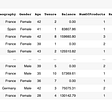## AI/ML training & Logging with Azure Databricks using AZURE MLFlow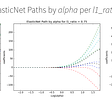## Confusion Matrix or its two types of error.## The dumb reason your fancy Computer Vision app isn’t working: Exif Orientation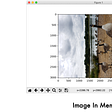## Is Die Hard a Christmas Movie? Let’s ask Azure!## Introducing AutoDist | Petuum## Ahmed Taha

I write reviews on computer vision papers. Writing tips are welcomed.

## Review — Billion-Scale Semi-Supervised Learning for Image Classification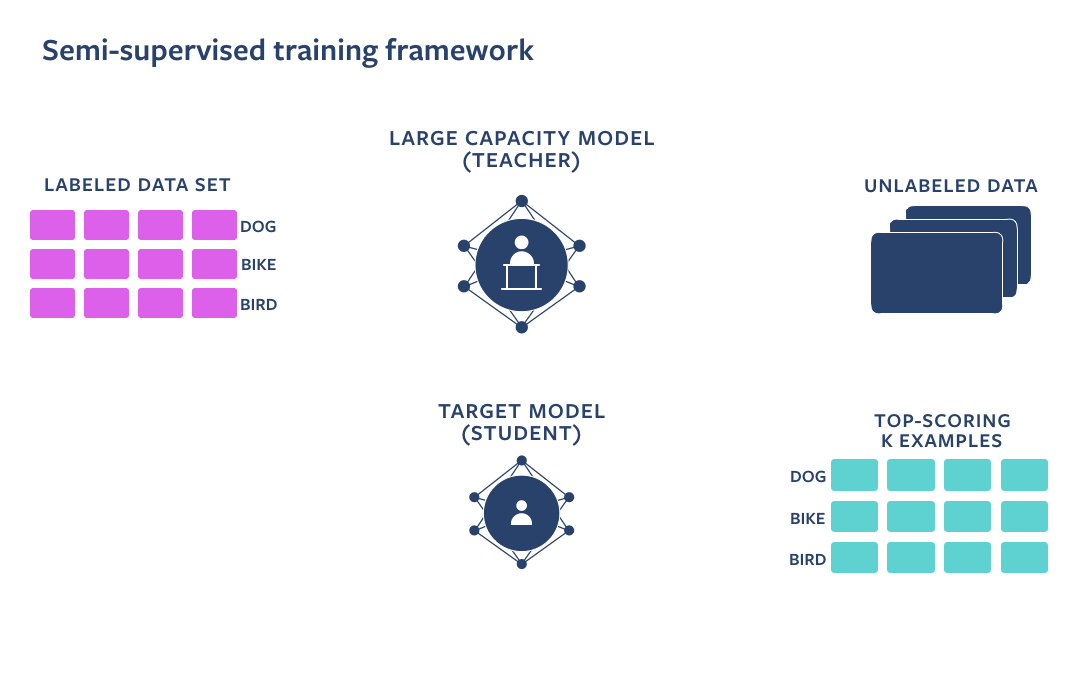## Autoencoders Demystified: Audio signal denoising## Pruning for Deep Neural Networks — Techniques to Prune Image and Language Models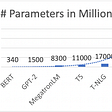## Paper Summary: Masked Autoencoders Are Scalable Vision Learners